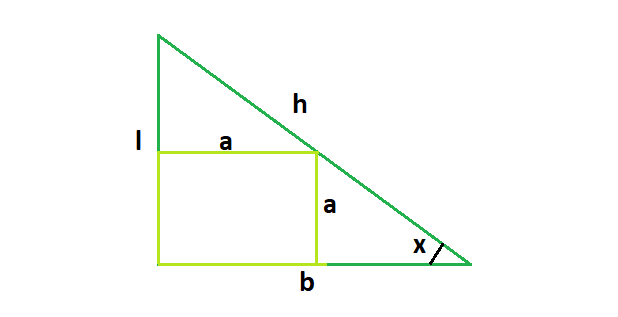# Area of a largest square fit in a right angle triangle

Given a right angled triangle with height l, base b & hypotenuse h.We need to find the area of the largest square that can fit in the right angled triangle.

Examples:

```Input: l = 3, b = 4, h = 5
Output: 2.93878
The biggest square that can fit inside
is of 1.71428 * 1.71428 dimension

Input: l = 5, b = 12, h = 13
Output: 12.4567
```

## Recommended: Please try your approach on {IDE} first, before moving on to the solution.Considering the above diagram, we see,tanx = l/b.
Here it is also true that, tanx = a/(b-a).
So, l/b = a/(b-a) which means that, a = (l*b)/(l+b)

Below is the required implementation:

## C++

 `// C++ Program to find the area of the biggest square ` `// which can fit inside the right angled traingle ` `#include ` `using` `namespace` `std; ` ` `  `// Function to find the area of the biggest square ` `float` `squareArea(``float` `l, ``float` `b, ``float` `h) ` `{ ` ` `  `    ``// the height or base or hypotenuse ` `    ``// cannot be negative ` `    ``if` `(l < 0 || b < 0 || h < 0) ` `        ``return` `-1; ` ` `  `    ``// side of the square ` `    ``float` `a = (l * b) / (l + b); ` ` `  `    ``// squaring to get the area ` `    ``return` `a * a; ` `} ` ` `  `// Driver code ` `int` `main() ` `{ ` `    ``float` `l = 5, b = 12, h = 13; ` `    ``cout << squareArea(l, b, h) << endl; ` ` `  `    ``return` `0; ` `} `

## Java

 `//Java Program to find the area of the biggest square ` `//which can fit inside the right angled traingle ` `public` `class` `GFG { ` ` `  `    ``//Function to find the area of the biggest square ` `    ``static` `float` `squareArea(``float` `l, ``float` `b, ``float` `h) ` `    ``{ ` ` `  `     ``// the height or base or hypotenuse ` `     ``// cannot be negative ` `     ``if` `(l < ``0` `|| b < ``0` `|| h < ``0``) ` `         ``return` `-``1``; ` ` `  `     ``// side of the square ` `     ``float` `a = (l * b) / (l + b); ` ` `  `     ``// squaring to get the area ` `     ``return` `a * a; ` `    ``} ` ` `  `    ``//Driver code ` `    ``public` `static` `void` `main(String[] args) { ` `         `  `         ``float` `l = ``5``, b = ``12``, h = ``13``; ` `         ``System.out.println(squareArea(l, b, h)); ` `    ``} ` `} `

## Python 3

 `# Python 3 Program  to find the  ` `# area of the biggest square  ` `# which can fit inside the right ` `# angled traingle  ` ` `  `# Function to find the area of the biggest square ` `def` `squareArea(l, b, h) : ` ` `  `    ``# the height or base or hypotenuse  ` `    ``# cannot be negative  ` `    ``if` `l < ``0` `or` `b < ``0` `or` `h < ``0` `: ` `        ``return` `-``1` ` `  `    ``# side of the square ` `    ``a ``=` `(l ``*` `b) ``/` `(l ``+` `b) ` ` `  `    ``# squaring to get the area ` `    ``return` `a ``*` `a ` ` `  `# Driver Code ` `if` `__name__ ``=``=` `"__main__"` `: ` ` `  `    ``l, b, h ``=` `5``, ``12``, ``13` ` `  `    ``print``(``round``(squareArea(l, b, h),``4``)) ` ` `  `# This code is contributed by ANKITRAI1 `

## C#

 `// C# Program to find the area of  ` `// the biggest square which can  ` `// fit inside the right angled triangle ` `using` `System; ` `class` `GFG  ` `{ ` ` `  `// Function to find the area  ` `// of the biggest square ` `static` `float` `squareArea(``float` `l, ``float` `b,  ` `                        ``float` `h) ` `{ ` ` `  `// the height or base or hypotenuse ` `// cannot be negative ` `if` `(l < 0 || b < 0 || h < 0) ` `    ``return` `-1; ` ` `  `// side of the square ` `float` `a = (l * b) / (l + b); ` ` `  `// squaring to get the area ` `return` `a * a; ` `} ` ` `  `// Driver code ` `public` `static` `void` `Main()  ` `{ ` `    ``float` `l = 5, b = 12, h = 13; ` `    ``Console.WriteLine(squareArea(l, b, h)); ` `} ` `} ` ` `  `// This code is contributed  ` `// by inder_verma.. `

## PHP

 ` `

Output:

```12.4567
```

My Personal Notes arrow_drop_upCheck out this Author's contributed articles.

If you like GeeksforGeeks and would like to contribute, you can also write an article using contribute.geeksforgeeks.org or mail your article to contribute@geeksforgeeks.org. See your article appearing on the GeeksforGeeks main page and help other Geeks.

Please Improve this article if you find anything incorrect by clicking on the "Improve Article" button below.

Article Tags :
Practice Tags :

Be the First to upvote.

Please write to us at contribute@geeksforgeeks.org to report any issue with the above content.